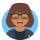Home
IT Knowledge
Inspiration
Languages
EN

# React - destructuring props (different ways)

0 points
Created by:Lily
208

In this article, we would like to show you how to use destructuring in React.

To simplify the work with objects (e.g. `props` object), we can use JavaScript ES6 functionality called destructuring. It consists of the fact that instead of extracting the values from individual keys to individual variables one by one, we can do it in bulk during one operation.

Take a look at the example below:

```
```const Component1 = (props) => {
const firstProperty = props.firstProperty;
const secondProperty = props.secondProperty;
// some code...
};``````

And now, after using destructuring:

```
```const Component2 = (props) => {
const { firstProperty: firstAlternativeVariable, secondProperty: secondAlternativeVariable} = props;
// some code...
};``````

On the right side of the equal sign, we insert the entire object that we will destructure. On the left, we build the shape of this object, i.e. we enter the keys that interest us, and then we declare constants with any names to which the values under these keys will be assigned.

### The syntax above is ok, but let's simplify it...

When our constants (or variables) are named the same as the keys under which we extract the values, we can use a simplified syntax - do not give the names of these keys - React will guess what they will be.

```
```const Component3 = (props) => {
const { firstProperty, secondProperty } = props;
// some code...
};``````

If we don't use the props object for anything other than to extract a value, then we can simplify it even more and not call the argument at all and do destructuring already in the parentheses at the beginning:

```
```const Component4 = ({ firstProperty, secondProperty }) => {
// some code...
};``````

Using this syntax, we can additionally assign default values to props.

``````const Component5 = ({ firstProperty = 'defaultValue1', secondProperty = 'defaultValue2' }) => {
// some code...
};``````

### Now let's check if what I wrote is true.

```
```// ONLINE-RUNNER:browser;

// Note: Uncomment import lines during working with JSX Compiler.
// import React from "react";
// import ReactDOM from "react-dom";

const Component1 = (props) => {
const name = props.name;
const surname = props.surname;
return <div>{name} {surname}</div>
};

const Component2 = (props) => {
const { name: alternativeName, surname: alternativeSurname } = props;
return <div>{alternativeName} {alternativeSurname}</div>
};

const Component3 = (props) => {
const { name, surname } = props;
return <div>{name} {surname}</div>
};

const Component4 = ({ name, surname }) => {
return <div>{name} {surname}</div>
};

const Component5 = ({ name = 'Bruce', surname = 'Banner' }) => {
return <div>{name} {surname}</div>
};

const App = () => {
const exampleObject = {
name: "John",
surname: "Wick"
}
return (
<div>
<Component1 {...exampleObject} />
<Component2 {...exampleObject} />
<Component3 {...exampleObject} />
<Component4 {...exampleObject} />
<Component5 />
</div>
);
};

const root = document.querySelector('#root');
ReactDOM.render(<App />, root);``````

Note:

In the above code, we use the `{... exampleObject}` expression - about this and other ways of passing objects as props we wrote here.